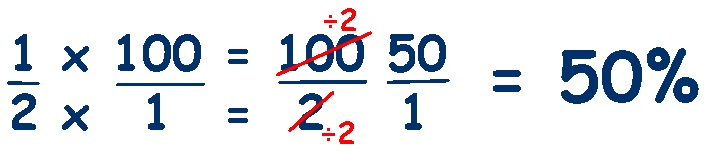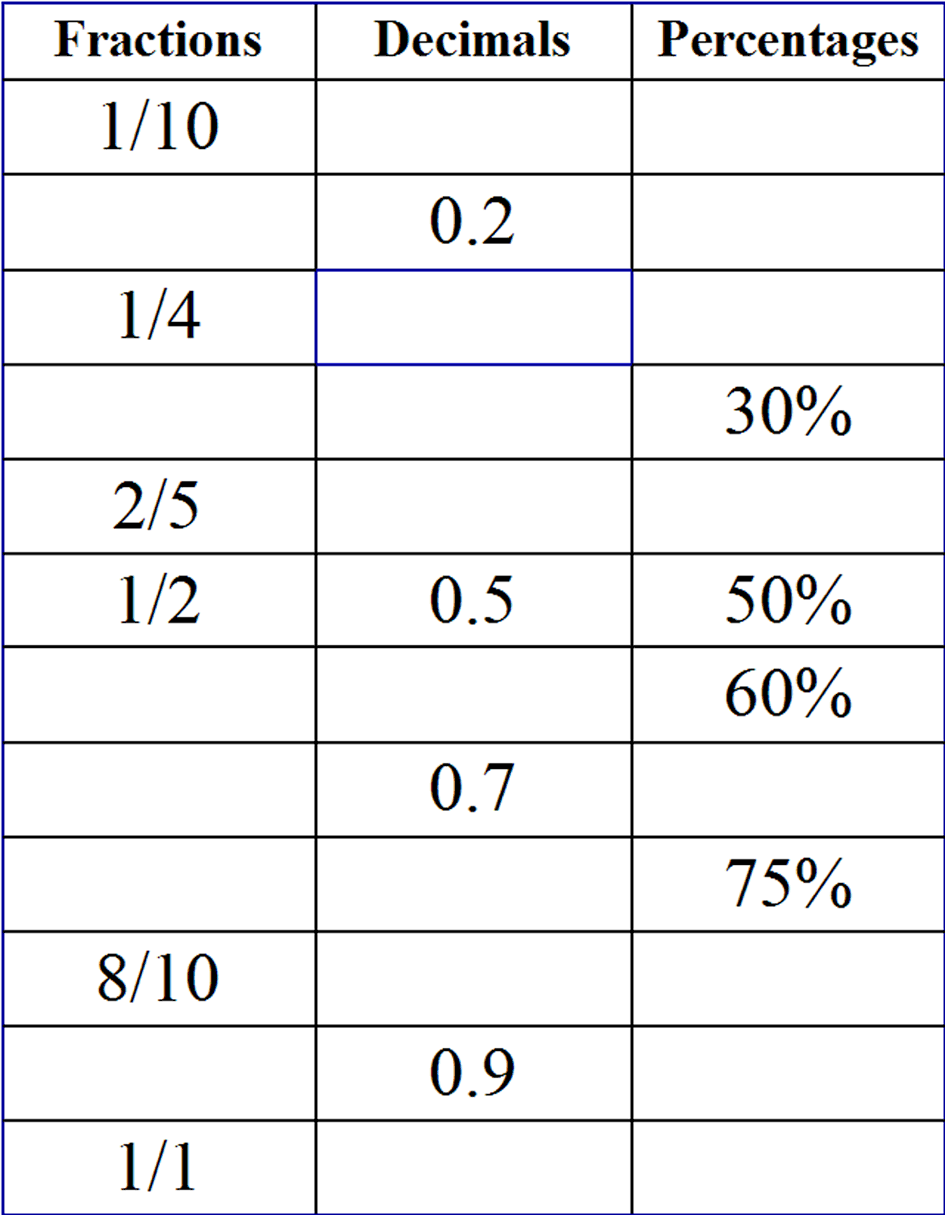# Percentage, Decimals and Fractions

### Percentages, Decimals and Fractions are all methods of showing the value of a part of the the total amount.

Your child needs to be able to convert easily between percentages, fractions and decimals as there are often questions which required this knowledge.

Lets look at a value that your child is familiar with, a half.

A half is an amount that is divided by two - it is 1 divided by 2 and represented as a fraction by 1/2.

We can convert fractions to decimals by dividing the numerator by the denominator,

### 1 ÷ 2 = 0.5 therefore 1/2 is represented as a decimal by 0.5

To find a percentage, we can simply multiply the fraction or the decimal by 100.

### The decimal value of 1/2 is 0.5 ... 0.5 x 100 = 50%

To multiply a fraction by 100 as a fraction, we need to convert 100 into a fraction,

which is 100/1 (any whole number as a fraction is itself divided by 1)

To multiply fractions we multiply the numerators and multiply the denominators,## so 1/2 = 0.5 = 50%

Practice converting percentages, decimals and fractions by completing this chartclick on chart to download a printable version

Try these resources from Mathsblog

Yr5 Percentages
Yr6 Percentages

and

Word Problems from Primary Resources

Test your knowledge of percentage with this activity from BBC Bitesize